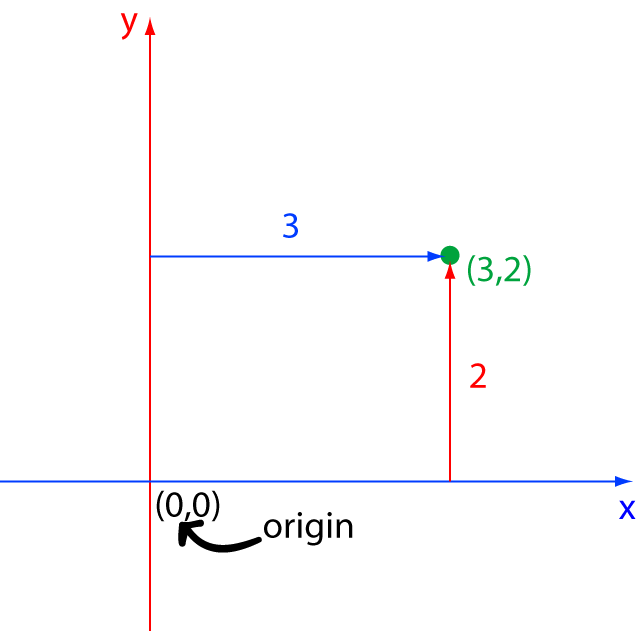# Definition of OriginThe origin is the starting point from which other numbers or points are measured.

The origin in a number line is the number $0$. The origin in the $xy$-plane is the point $(0,0)$.

To write the origin in higher dimensional spaces, add an extra zero. So the origin in $3$-dimensional space is the point $(0,0,0)$.

We often use the letter $O$ to denote the origin.

### Description

The aim of this dictionary is to provide definitions to common mathematical terms. Students learn a new math skill every week at school, sometimes just before they start a new skill, if they want to look at what a specific term means, this is where this dictionary will become handy and a go-to guide for a student.

### Audience

Year 1 to Year 12 students

### Learning Objectives

Learn common math terms starting with letter O

Author: Subject Coach
You must be logged in as Student to ask a Question.